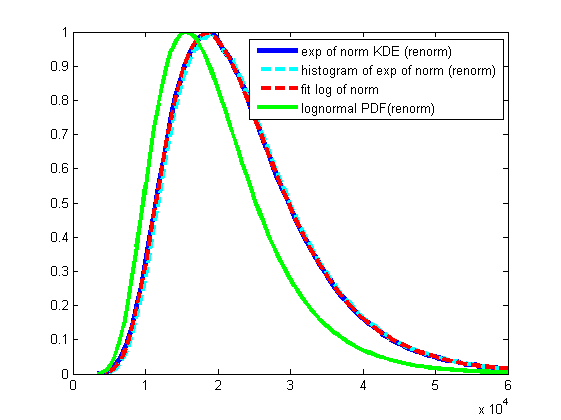PDF of LogNormal distribution from random numbers

katpyxa

New Member
Dear all,

I have trouble understanding why "textbook" formula for probability density function (PDF) of lognormal distribution is different from results I got when I try to generate PDF using random data.

So here is classic formula of lognormal PDF from Wikipediawith 1/x before exponent.

Wikipedia says that if Y is distributed normally then Z=exp(Y) is distributed lognormally.
Ok, let's verify this statement.
Using matlab I've generated 1000000 random samples from normal distribution with mean=10 and SD=0.4 and then for each value I've calculated its exp (getting another 1000000) and plotted resulting PDF of those, see here:I rescaled all functions in Y, so I can compare them, it should be fine.
So the blue line is PDF of exponent of this normal distribution using kernel density estimation and in dashed cyan is just plain histogram.

What confuses me (and this is my main question) is that if I plot PDF of lognormal distribution with the same parameters for mean and SD (in green) according to formula above, I got different shape of PDF.
Why is it so?
If I remove 1/x from pre-exponential term then PDF (dashed red) fits PDF generated from random samples perfectly.
Also if I multiply generated PDF by 1/x, it can be fitted by "textbook" formula.

My follow-up question is this. If I observe some process which is normal, but I observe it through device that "exponents" measurements. What function should I fit to the generated PDF?

I'm sorry if this question was already asked, I've googled and checked forums, but cannot find the answer, just point me there, thanks.

Dason

It's not clear to me what you did. Can you post the code you used to create your plot.